Skip to main content

# A novel fractional-order hyperchaotic system with a quadratic exponential nonlinear term and its synchronization

## Abstract

The dynamics of fractional-order systems have attracted increasing attention in recent years. In this paper a novel fractional-order hyperchaotic system with a quadratic exponential nonlinear term is proposed and the synchronization of a new fractional-order hyperchaotic system is discussed. The proposed system is also shown to exhibit hyperchaos for orders 0.95. Based on the stability theory of fractional-order systems, the generalized backstepping method (GBM) is implemented to give the approximate solution for the fractional-order error system of the two new fractional-order hyperchaotic systems. This method is called GBM because of its similarity to backstepping method and more applications in systems than it. Generalized backstepping method approach consists of parameters which accept positive values. The system responses differently for each value. It is necessary to select proper parameters to obtain a good response because the improper selection of parameters leads to inappropriate responses or even may lead to instability of the system. Genetic algorithm (GA), cuckoo optimization algorithm (COA), particle swarm optimization algorithm (PSO) and imperialist competitive algorithm (ICA) are used to compute the optimal parameters for the generalized backstepping controller. These algorithms can select appropriate and optimal values for the parameters. These minimize the cost function, so the optimal values for the parameters will be found. The selected cost function is defined to minimize the least square errors. The cost function enforces the system errors to decay to zero rapidly. Numerical simulation results are presented to show the effectiveness of the proposed method.

## 1 Introduction

Chaos synchronization has attracted a great deal of attention since Pecora and Carroll  established a chaos synchronization scheme for two identical chaotic systems with different initial conditions. Various effective methods such as robust control , the sliding method control , linear and nonlinear feedback control , function projective , adaptive control , active control , backstepping control , generalized backstepping method control  and anti-synchronization  have been presented to synchronize various chaotic systems.

The history of fractional calculus is more than three centuries old. It was found that the behavior of many physical systems can be properly described by fractional-order systems. Nowadays, it has been found that some fractional-order differential systems such as the fractional-order jerk model , the fractional-order Lorenz system , the fractional-order Chen system , the fractional-order Lu system , the fractional-order Rossler system , the fractional-order Arneodo system , the fractional-order Chua circuit , the fractional-order Duffing system  and the fractional-order Newton-Leipnik system  can demonstrate chaotic behavior. Due to their potential applications in secure communication and control processing, the fractional-order chaotic systems have been studied extensively in recent years in many aspects such as chaotic phenomena, chaotic control, chaotic synchronization and other related studies.

Recently, chaos synchronization problems in fractional-order systems have been widely investigated. For example, the synchronization of fractional-order chaotic systems utilized feedback control method , activation feedback control , robust control . The hybrid projective synchronization of different dimensional fractional order chaotic systems was investigated in . Synchronization between two fractional-order systems was achieved by utilizing a single-variable feedback method . In  the author utilized active control technique to synchronize different fractional-order chaotic dynamical systems. A novel active pinning control strategy was utilized for synchronization and anti-synchronization of new uncertain fractional-order unified chaotic systems (UFOUCS) .  investigated the function projective synchronization between fractional-order chaotic systems. In  the synchronization of N-coupled fractional-order chaotic systems with ring connection was first firstly investigated in detail. A method to achieve general projective synchronization of two fractional-order Rossler systems was proposed in . In , the fractional-order Rossler system was synchronized by active control method. In this work, we investigate a novel fractional-order hyperchaotic system with a quadratic exponential nonlinear term and its synchronization.

The rest of the paper is organized as follows. In Section 2, the definition of fractional-order derivative and its approximation is presented. In Section 3, a novel fractional-order hyperchaotic system is presented. In Section 4, the generalized backstepping method is described. In Section 5, synchronization between two novel fractional-order hyperchaotic systems is achieved by generalized backstepping method. In Section 6, the designed controller is optimized by evolutionary algorithms. Section 7 presents, simulation results. Finally, Section 8 provides, conclusion of this work.

## 2 Fractional-order derivative and its approximation

To discuss fractional chaotic systems, we usually need to solve fractional-order differential equations. For the fractional differential operator, there are two commonly used definitions: Grünwald-Letnikov (GL) definition and Riemann-Liouville (RL) definition. The GL definition of non-integer integration and differentiation is given as follows [33, 34]:

$D t α f(t)= lim h → ∞ h − α ∑ j = 0 [ ( t − α ) / h ] ( − 1 ) j ( α j ) f(t−jh),$
(1)

where $( α j ) = α ( α − 1 ) ⋯ ( α − j + 1 ) j !$. This formula can be reduced to

$D t α y( t m )≈ h − α ∑ j = 0 m ω j α y m − j ,$
(2)

where $ω j α = ( − 1 ) j ( α j )$ ($j=0,1,2,…$) and h is the time step. The best-known RL definition of fractional-order, described by  is as follows:

$d α f ( t ) d t α = 1 Γ ( n − α ) d n d t n 0 t f ( τ ) ( t − τ ) α − n + 1 dτ,$
(3)

where n is an integer such that $n−1≤α, $Γ(⋅)$ is the Γ-function. The Laplace transform of the Riemann-Liouville fractional derivative is

$L { d α f ( t ) d t α } = s α L { f ( t ) } − ∑ k = 0 n − 1 s k [ d α − 1 − k f ( t ) d t α − 1 − k ] t = 0 ,$
(4)

where, L means the Laplace transform and s is a complex variable. Upon considering the initial conditions to zero, this formula reduces to

$L { d α f ( t ) d t α } = s α L { f ( t ) } .$
(5)

Thus, the fractional integral operator of order α can be represented by the transfer function $H(s)= 1 s α$ in the frequency domain . The standard definitions of fractional-order calculus do not allow direct implementation of the fractional operators in time-domain simulations. An efficient method to circumvent this problem is to approximate fractional operators by using standard integer-order operators. In Ref. , an effective algorithm is developed to approximate fractional-order transfer functions, which has been adopted in  and has sufficient accuracy for time-domain implementations. We will use the $1 s 0.95$ approximation formula  in the following simulation examples:

$1 s 0.95 ≈ 1.2831 s 2 + 18.6004 s + 2.0833 s 3 + 18.4738 s 2 + 2.6574 s + 0.003 .$
(6)

The $1 s 0.95$ approximation formula has a similar theoretical basis as the above analysis.

## 3 System description

Recently, Fei Yu and Chunhua Wang constructed the 3D autonomous chaotic system with a quadratic exponential nonlinear term . The system is described by

$x ˙ = a ( y − x ) , y ˙ = b x − c x z , z ˙ = e x y − d z ,$
(7)

where a, b, c, d are positive constants and x, y, z are variables of the system, when $a=10$, $b=40$, $c=2$, $d=2.5$, system (7) is chaotic. See Figure 1.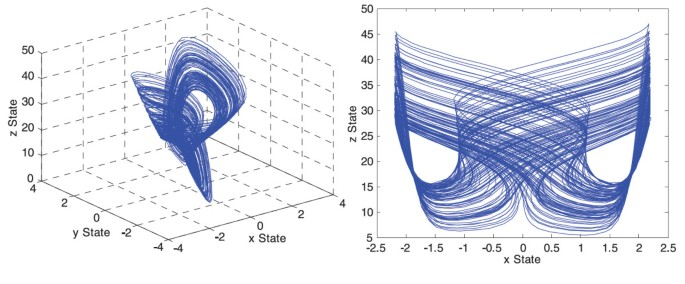Figure 1

Here, the reverse structure form of system (7) is described by

$x ˙ = a ( y − x ) , y ˙ = b x + c x z , z ˙ = − e x y − d z .$
(8)

Obviously, in system (8) the sign of the multiplier in the second equation is positive and the sign of the quadratic exponential term in the third equation is negative, which is the only difference between system (8) and system (7). Similarly, when $a=10$, $b=40$, $c=2$, $d=2.5$, the Lyapunov exponents of this system are obtained to be $L 1 =1.389$, $L 2 =0$, $L 3 =−13.866$ by Wolf method . And the Lyapunov dimension is $D L =2.1002$. Apparently, system (8) is also a chaotic system. The strange attractors of system (8) are shown in Figure 2. From the figure, we can see the strange attractor is a reverse butterfly-shape attractor. Therefore, system (8) is a reverse structure system (7).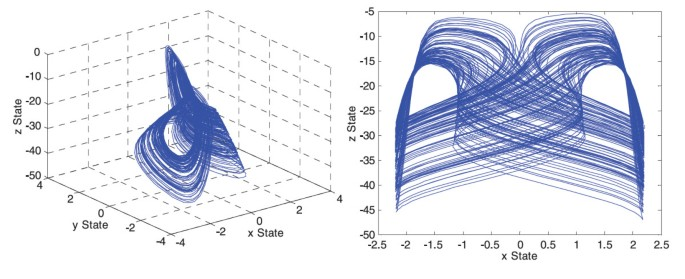Figure 2

In order to obtain hyperchaos, we introduce an additional state w to system (8), and add it to the first and second equations of system (8). Then we get the following 4D system: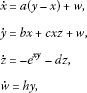(9)

where a, b, c, d are positive parameters of system (8) and h is a parameter to be determined, its value can be varied within a certain range. When parameters $a=10$, $b=40$, $c=2$, $d=2.5$ and $h=−4$, the four Lyapunov exponents of system (9) are $L 1 =1.019$, $L 2 =0.367$, $L 3 =0$, $L 4 =−13.851$. The Lyapunov exponent spectrum of new chaotic system (9) is shown in Figure 3. The Lyapunov dimension of system (9) is given as follows:

$D L =j+ ∑ i = 1 j L i | L j + 1 | =3+ 1.019 + 0.367 | − 13.851 | =3.1001.$
(10)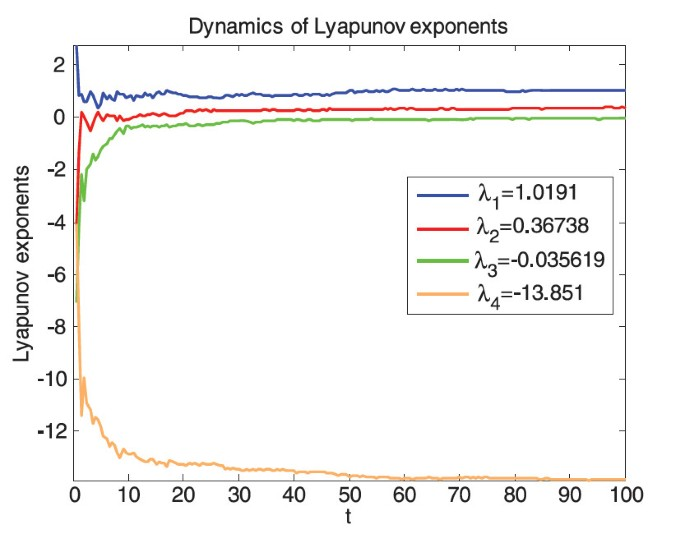Figure 3

Therefore, system (9) with the parameter $h=−4$ shows hyperchaotic behavior. The hyperchaotic attractor is given in Figure 4.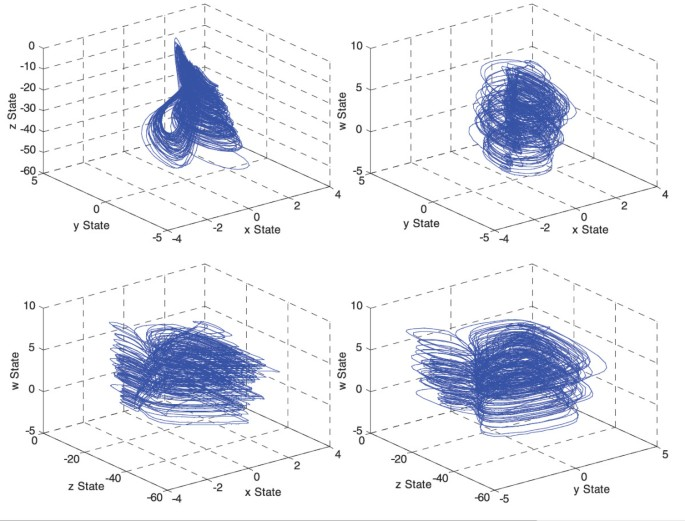Figure 4

The new fractional-order hyperchaotic system is given as follows:

$d q x d t q = a ( y − x ) + w , d q y d t q = b x + c x z + w , d q z d t q = − e x y − d z , d q w d t q = h y .$
(11)

Here, $a=10$, $b=40$, $c=2$, $d=2.5$ and $h=−4$, where q is the fractional order. System (11) exhibits chaotic attractor; see Figure 5. In the following, we choose $q=0.95$.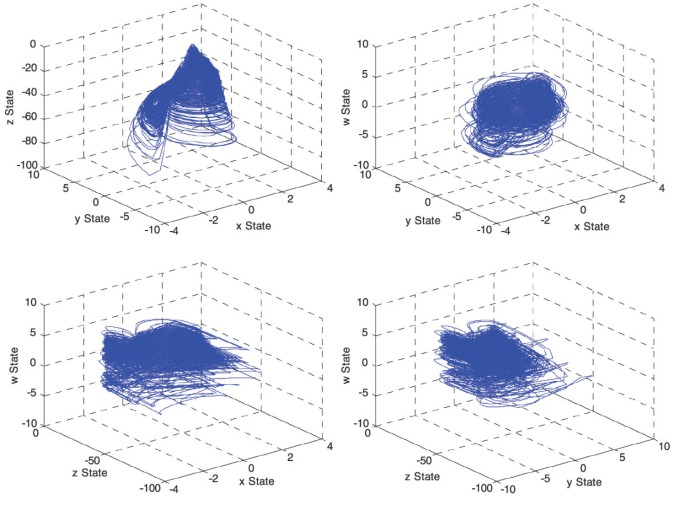Figure 5

## 4 The generalized backstepping method

Generalized backstepping method (GBM)  will be applied to a certain class of autonomous nonlinear systems which are expressed as follows:

${ X ˙ = F ( X ) + G ( X ) η , η ˙ = f 0 ( X , η ) + g 0 ( X , η ) u ,$
(12)

in which $η∈R$ and $x=[ x 1 , x 2 ,…, x n ]∈R$. In order to obtain an approach to control these systems, we may need to prove a new theorem as follows.

Theorem Suppose equation (12) is available, then suppose the scalar function $φ i (x)$ for the ith state could be determined by inserting the ith term for η, the function $V(x)$ would be a positive definite equation (13) with negative definite derivative:

$V(X)= 1 2 ∑ i = 1 n x i 2 .$
(13)

Therefore, the control signal and also the general control Lyapunov function of this system can be obtained by equations (14), (15):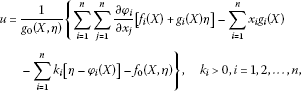(14)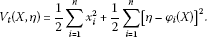(15)

Proof Equation (12) can be represented as the extended form of equation (16):

${ x ˙ i = f i ( X ) + g i ( X ) η ; i = 1 , 2 , … , n , η ˙ = f 0 ( X , η ) + g 0 ( X , η ) u .$
(16)

$V(X)$ is always positive definite, and therefore the negative definite of its derivative should be examined; it means $W(X)$ in equation (17) should always be positive definite so that $V ˙ (X)$ would be negative definite.

$V ˙ (X)= ∑ i = 1 n x i x ˙ i = ∑ i = 1 n x i [ f i ( X ) + g i ( X ) φ i ( X ) ] ≤−W(X).$
(17)

By $u 0 = f 0 (X,η)+ g 0 (X,η)u$ and adding and subtracting $g i (X) φ i (X)$ to the i th term of (16), (18) be obtained

${ x ˙ i = [ f i ( X ) + g i ( X ) φ i ( X ) ] + g i ( X ) [ η − φ i ( X ) ] , η ˙ = u 0 ; i = 1 , 2 , … , n .$
(18)

Now, we use the following change of a variable: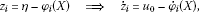(19)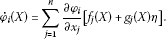(20)

Therefore, equation (18) would be obtained as follows:

${ x ˙ i = [ f i ( X ) + g i ( X ) φ i ( X ) ] + g i ( X ) [ η − φ i ( X ) ] , z ˙ i = u 0 − φ ˙ i ( X ) ; i = 1 , 2 , … , n .$
(21)

Regarding that $z i$ has n states, then $u 0$ can be considered with n terms provided that equation (22) would be established as follows:

$u 0 = ∑ i = 1 n u i .$
(22)

Therefore, the last term of equation (21) would be converted to equation (23):

$z ˙ i = u i − φ ˙ i (X)= λ i .$
(23)

At this stage, the control Lyapunov function would be considered as equation (24):

$V t (X,η)= 1 2 ∑ i = 1 n x i 2 + 1 2 ∑ i = 1 n z i 2$
(24)

which is a positive definite function. Now, it is sufficient to examine negative definitely of its derivative

$V ˙ t (X,η)= ∑ i = 1 n ∂ V ( X ) ∂ x i [ f i ( X ) + g i ( X ) φ i ( X ) ] + ∑ i = 1 n ∂ V ( X ) ∂ x i g i (X)+ ∑ i = 1 n z i λ i .$
(25)

In order that the function $V ˙ t (X,η)$ would be negative definite, it is sufficient that the value of $λ i$ would be selected as equation (26)

$λ i =− ∂ V ( X ) ∂ x i g i (X)− k i z i ; k i >0.$
(26)

Therefore, the value of $V ˙ t (X,η)$ would be obtained from following equation:

$V ˙ t (X,η)= ∑ i = 1 n x i [ f i ( X ) + g i ( X ) φ i ( X ) ] − ∑ i = 1 n k i z i 2 ≤−W(X)− ∑ i = 1 n k i z i 2$
(27)

which indicates negative finitely status of the function $V ˙ t (X,η)$. Consequently, the control signal function, using equations (18), (20) and (21) would be converted to equation (26)

$u 0 = ∑ i = 1 n ∑ j = 1 n ∂ φ i ∂ x j [ f j ( X ) + g j ( X ) η ] − ∑ i = 1 n x i g i (X)− ∑ i = 1 n k i [ η − φ i ( X ) ] .$
(28)

Therefore, using the variations of the variables which we carried out, equations (14), (15) can be obtained. Now, considering the unlimited region of positive definitely of $V t (X,η)$ and negative definitely of $V ˙ t (X,η)$ and the radially unbounded space of its states, global stability gives the proof. □

## 5 Synchronization between the two novel fractional order hyperchaotic systems

In order to achieve the behavior of synchronization between two new chaotic systems by using the proposed method, suppose the drive system takes the following from:

$d q x 1 d t q = a ( y 1 − x 1 ) + w 1 , d q y 1 d t q = b x 1 + c x 1 z 1 + w 1 , d q z 1 d t q = − e x 1 y 1 − d z 1 , d q w 1 d t q = h y 1 .$
(29)

And the response system is given as follows:

$d q x 2 d t q = a ( y 2 − x 2 ) + w 2 + u 1 ( t ) , d q y 2 d t q = b x 2 + c x 2 z 2 + w 2 + u 2 ( t ) , d q z 2 d t q = − e x 2 y 2 − d z 2 + u 3 ( t ) , d q w 2 d t q = h y 2 + u 4 ( t ) ,$
(30)

where $u 1 (t)$, $u 2 (t)$, $u 3 (t)$ and $u 4 (t)$ are control functions to be determined for achieving synchronization between two systems (29) and (30). Define state errors between systems (29) and (30) as follows:

$e x = x 2 − x 1 , e y = y 2 − y 1 , e z = z 2 − z 1 , e w = w 2 − w 1 .$
(31)

We obtain the following error dynamical system by subtracting drive system (29) from response system (30):

$d q e x d t q = a ( e y − e x ) + e w + u 1 , d q e y d t q = b e x + e w + c ( x 2 z 2 − x 1 z 1 ) + u 2 , d q e z d t q = − d e z + e x 1 y 1 − e x 2 y 2 + u 3 , d q e w d t q = h e y + u 4 .$
(32)

In order to determine the controller, let

$u 1 = 0 , u 2 = − c ( x 2 z 2 − x 1 z 1 ) , u 3 = e x 2 y 2 − e x 1 y 1 + v 3 , u 4 = v 4 ,$
(33)

where $v 3 (t)$ and $v 4 (t)$ are control inputs. Substituting equation (33) into equation (32) yields

$d q e x d t q = a ( e y − e x ) + e w , d q e y d t q = b e x + e w , d q e z d t q = − d e z + v 3 , d q e w d t q = h e y + v 4 .$
(34)

Thus, error system (34) is to be controlled with control inputs $v 3 (t)$ and $v 4 (t)$ as functions of error states $e x$, $e y$, $e z$ and $e w$. When system (34) is stabilized by control inputs $v 3 (t)$ and $v 4 (t)$, $e x$, $e y$, $e z$ and $e w$ will converge to zeros as time t tends to infinity, which. Implies that systems (29) and (30) are synchronized. In order to use the theorem, it is sufficient to establish equations (35) and (36):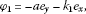(35)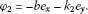(36)

According to the theorem, the control signals will be obtained from equations (37) and (38)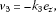(37)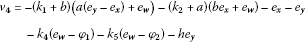(38)

and Lyapunov function as follows:

$V( e x , e y , e z , e w )= 1 2 e x 2 + 1 2 e y 2 + 1 2 e z 2 + 1 2 e w 2 + 1 2 ( e w − φ 1 ) 2 + 1 2 ( e w − φ 2 ) 2 .$
(39)

Error system (32) is reduced to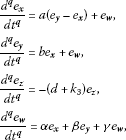(40)

where

${ α = ( a − k 4 ) k 1 − b ( k 2 + k 5 ) − 1 , β = − ( a ( k 1 + k 4 + b ) + k 2 k 5 + 1 ) , γ = − ( a + b + k 1 + k 2 + k 4 + k 5 ) , k 1 , k 2 , k 3 , k 4 , k 5 > 0 .$
(41)

Take the Laplace transformation on both sides of equation (40), let $E i (s)=L{ e i (t)}$ and utilize $L{ d q e i d t q }= s q E i (s)− s q − 1 e i (0)$ ($i=x,y,z,w$), then we obtain

${ s q E x ( s ) − s q − 1 e x ( 0 ) = a ( E y ( s ) − E x ( s ) ) + E w ( s ) , s q E y ( s ) − s q − 1 e y ( 0 ) = b E x ( s ) + E w ( s ) , s q E z ( s ) − s q − 1 e z ( 0 ) = − ( d + k 3 ) E z ( s ) , s q E w ( s ) − s q − 1 e w ( 0 ) = α E x ( s ) + β E y ( s ) + γ E w ( s ) .$
(42)

Its solution can be explicitly expressed as follows: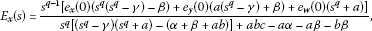(43)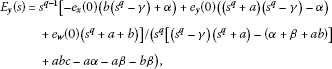(44)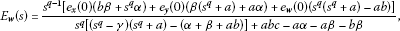(45)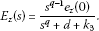(46)

According to the final value theorem of the Laplace transformation, considering the assumed conditions, we have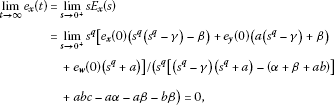(47)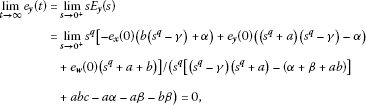(48)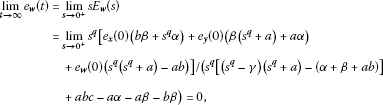(49)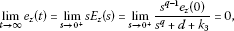(50)

where $abc−aα−aβ−bβ≠0$ and $d+ k 3 ≠0$. The above results manifest the novel fractional-order hyperchaotic systems (29) and (30) which are synchronized under the control law (33).

## 6 Optimization of generalized backstepping controller

The Genetic algorithm (see Table 1) [44, 45], cuckoo optimization algorithm (see Table 2) , particle swarm optimization algorithm (see Table 3)  and imperialist competitive algorithm (see Table 4)  are used to search the optimal parameter (k) in order to guarantee the stability of systems by ensuring negativity of the Lyapunov function and having a suitable time response. The controller in equation (33) is optimized by the cost function in equation (51)

$f( e 1 , e 2 ,…, e n )= 1 n ∑ i = 1 n e i 2 d t .$
(51)

## 7 Numerical simulation

This section presents synchronization of numerical simulations between two new fractional-order hyperchaotic systems. The generalized backstepping method (GBM) is used as an approach to synchronize the new fractional order hyperchaotic system. The initial values of drive and response systems are $x 1 (0)=2.2$, $y 1 (0)=2.4$, $z 1 (0)=−28$, $w 1 (0)=−20$ and $x 2 (0)=−2.2$, $y 2 (0)=−2.4$, $z 2 (0)=28$, $w 2 (0)=20$ respectively. The optimal parameters of generalized backstepping controller using genetic algorithm, cuckoo optimization algorithm, particle swarm optimization algorithm and imperialist competitive algorithm are listed in Table 5.

The time response of x, y, z, w states for drive system (29) and response system (30) via generalized backstepping method is shown in Figure 6 to Figure 9. Synchronization errors $( e x , e y , e z , e w )$ in new fractional-order hyperchaotic systems are shown in Figure 10 to Figure 13. The time response of the control inputs $( u 2 , u 3 , u 4 )$ for the synchronization of new fractional-order hyperchaotic systems is shown in Figure 14 to Figure 17.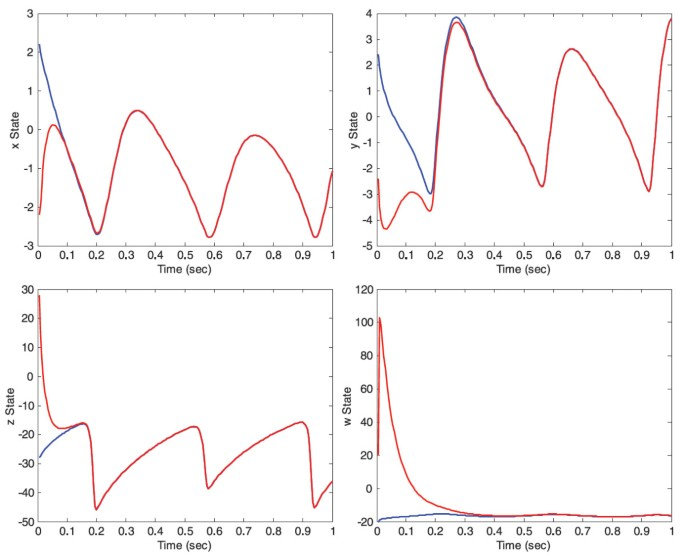Figure 6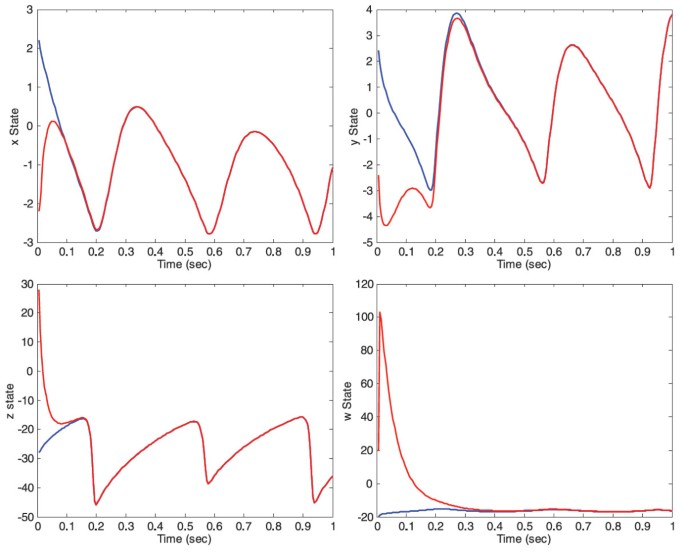Figure 7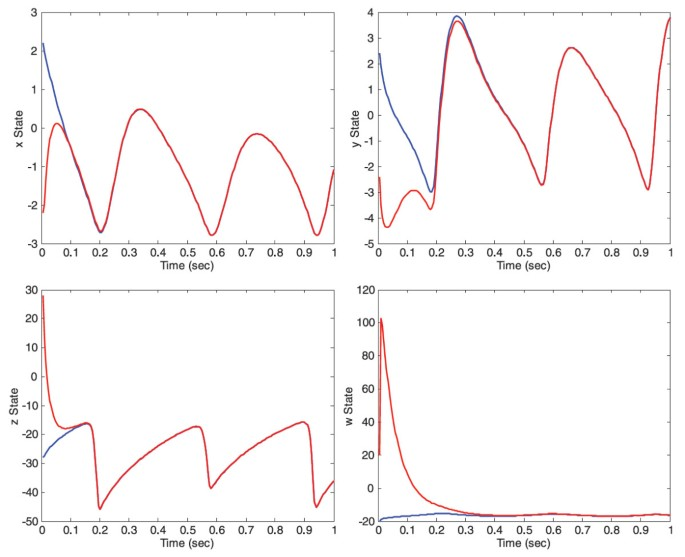Figure 8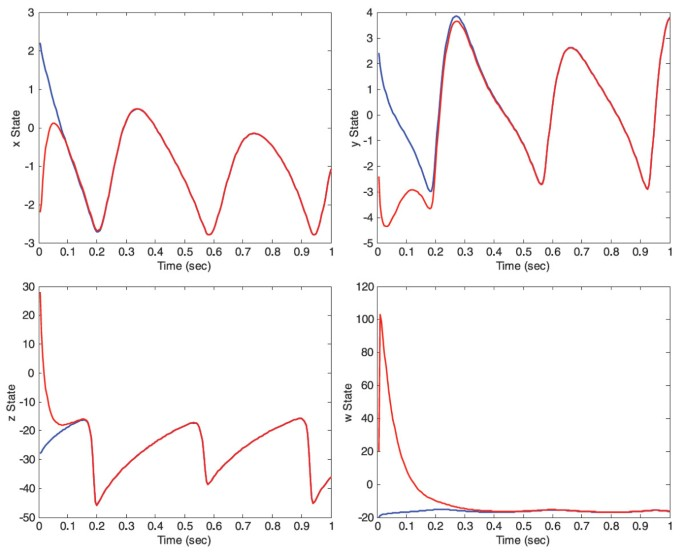Figure 9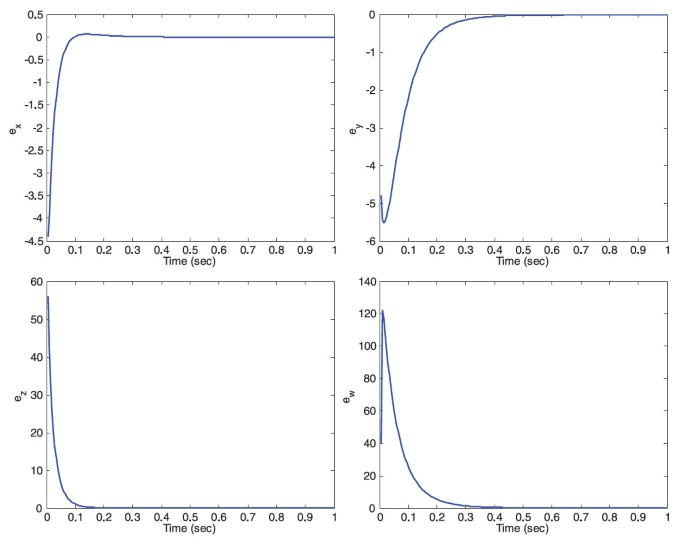Figure 10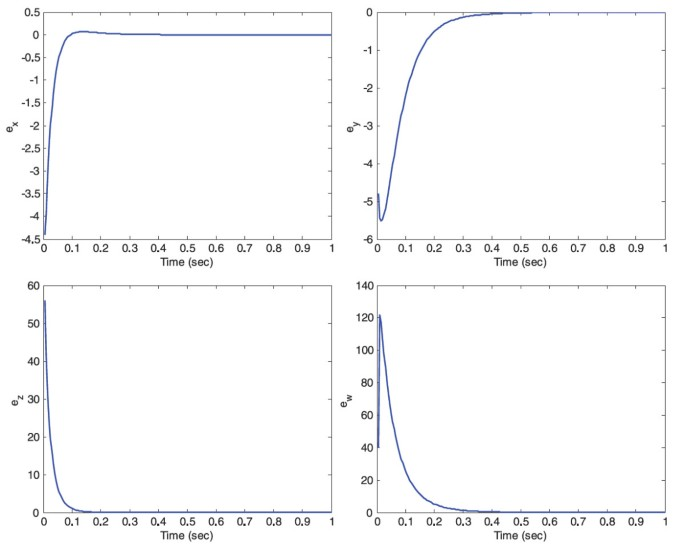Figure 11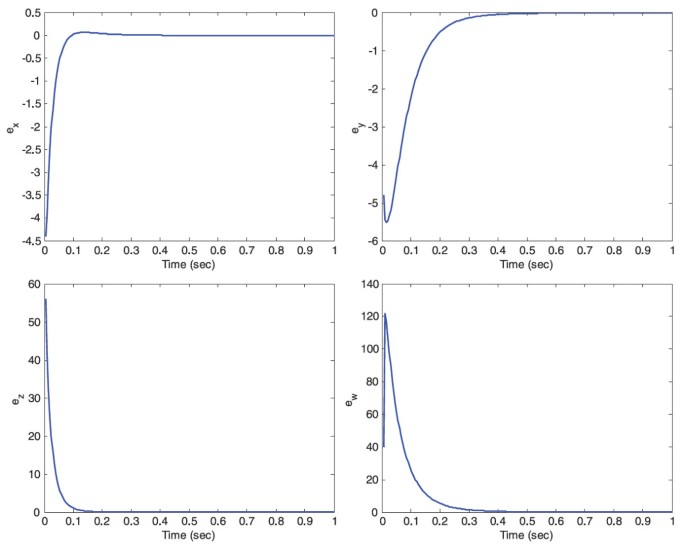Figure 12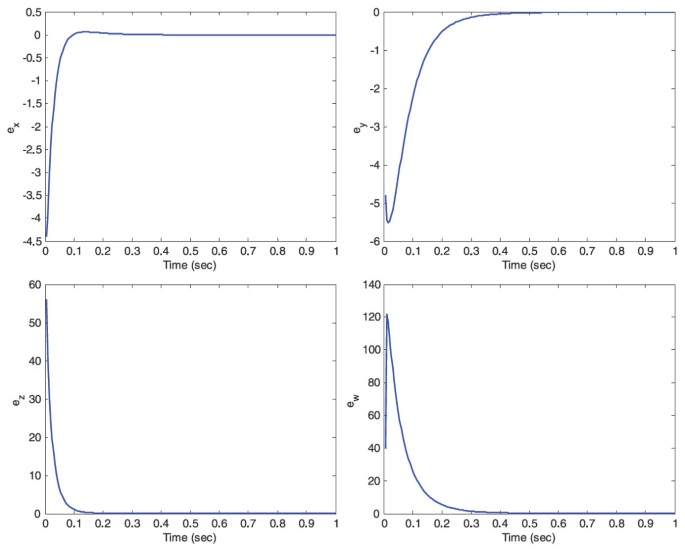Figure 13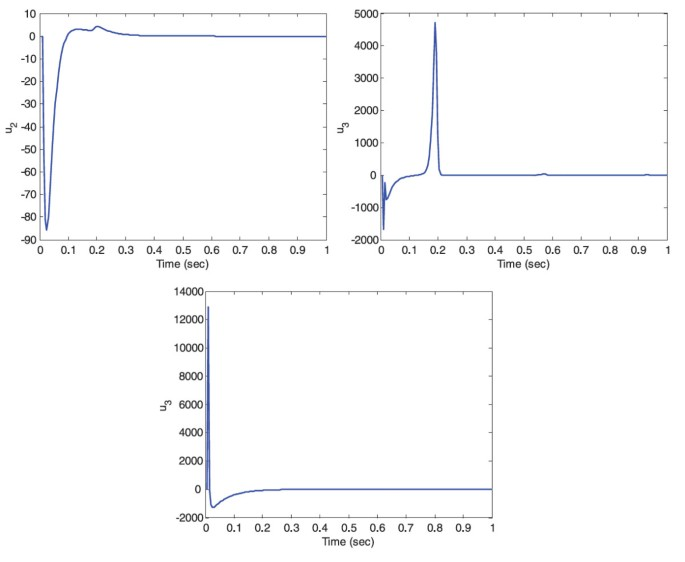Figure 14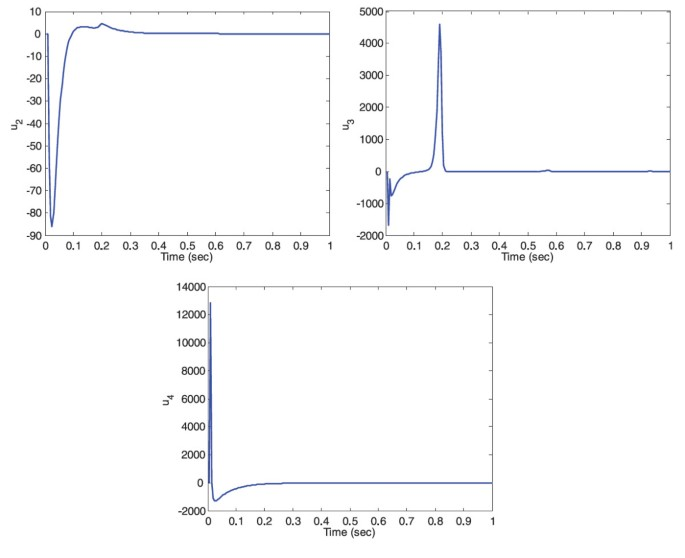Figure 15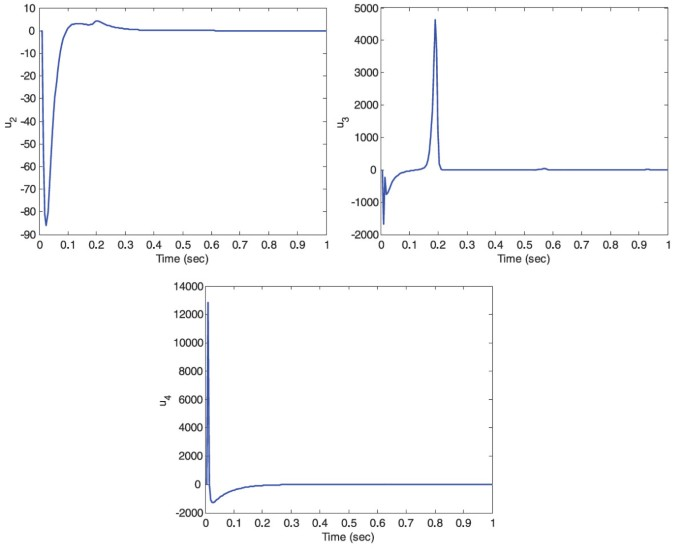Figure 16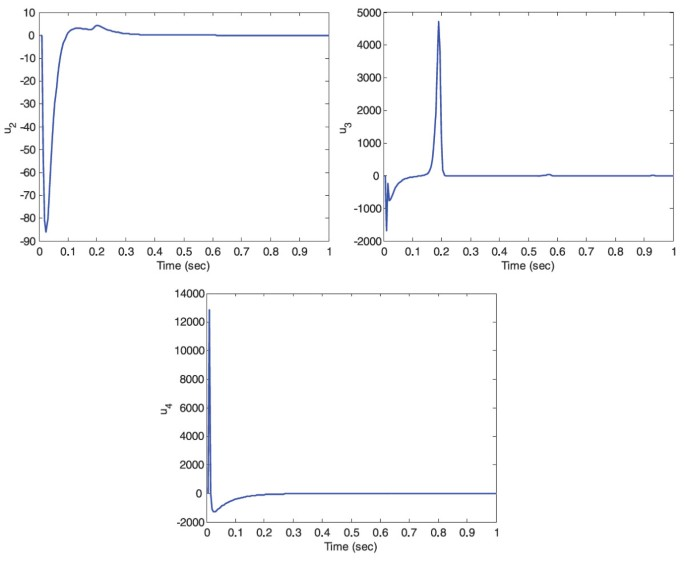Figure 17

## 8 Conclusions

In this work, the synchronization in a novel fractional-order hyperchaotic system with a quadratic exponential nonlinear term has been studied. This synchronization between two new fractional-order systems was achieved by generalized backstepping method. The designed controller consisted of parameters which accepted positive values. Improper selection of the parameters causes improper behavior which may cause serious problems such as instability of the system. It was needed to optimize these parameters. Evolutionary algorithms were well known optimization method. Genetic algorithm, cuckoo optimization algorithm, particle swarm optimization algorithm and imperialist competitive algorithm optimized the controller to gain optimal and proper values for the parameters. For this reason these algorithms minimized the cost function to find minimum current value for it. On the other hand, the cost function finds the minimum value to minimize least square errors. Finally, numerical simulation was given to verify the effectiveness of the proposed synchronization scheme.

## References

1. 1.

Pecora L, Carroll T: Synchronization in chaotic systems. Phys. Rev. Lett. 1990, 64(2):821–824.

2. 2.

Kebriaei H, Yazdanpanah MJ: Robust adaptive synchronization of different uncertain chaotic systems subject to input nonlinearity. Commun. Nonlinear Sci. Numer. Simul. 2010, 15: 430–441. 10.1016/j.cnsns.2009.04.005

3. 3.

Sundarapandian V, Sivaperumal S: Global chaos synchronization of the hyperchaotic Qi systems by sliding mode control. Int. J. Comput. Sci. Eng. 2011, 3(6):2430–2437.

4. 4.

Yang L-X, Chu Y-D, Zhang J-G, Li X-F, Chang Y-X: Chaos synchronization in autonomous chaotic system via hybrid feedback control. Chaos Solitons Fractals 2009, 41: 214–223. 10.1016/j.chaos.2007.11.032

5. 5.

Zheng S, Dong G, Bi Q: A new hyperchaotic system and its synchronization. Appl. Math. Comput. 2010, 215: 3192–3200. 10.1016/j.amc.2009.09.060

6. 6.

Wu R, Liu J: Isochronal function projective synchronization between chaotic and time-delayed chaotic systems. Adv. Differ. Equ. 2012., 2012: Article ID 37

7. 7.

Yu F, Wang C, Hu Y, Yin J: Projective synchronization of a five-term hyperbolic-type chaotic system with fully uncertain parameters. Acta Phys. Sin. 2012., 61(6): Article ID 060505

8. 8.

Sundarapandian V, Karthikeyan R: Global chaos synchronization of hyperchaotic Lorenz and hyperchaotic Chen systems by adaptive control. Int. J. Comput. Sci. Eng. 2011, 3(6):2458–2472.

9. 9.

Shahiri M, Ghaderi R, Ranjbar NA, Hosseinnia SH, Momani S: Chaotic fractional-order Coullet system: synchronization and control approach. Commun. Nonlinear Sci. Numer. Simul. 2010, 15: 665–674. 10.1016/j.cnsns.2009.05.054

10. 10.

Lin C-M, Peng Y-F, Lin M-H: CMAC-based adaptive backstepping synchronization of uncertain chaotic systems. Chaos Solitons Fractals 2009, 42: 981–988. 10.1016/j.chaos.2009.02.028

11. 11.

Sahab AR, Taleb Ziabari M: Adaptive generalization backstepping method to synchronize T-system. Recent Advances in Computers, Communications, Applied Social Science and Mathematics 2011.

12. 12.

Yu F, Wang C, Hu Y, Yin J: Antisynchronization of a novel hyperchaotic system with parameter mismatch and external disturbances. Pramana J. Phys. 2012. doi:10.1007/s12043–012–0285–6

13. 13.

Ahmad WM, Sprott JC: Chaos in fractional-order autonomous nonlinear systems. Chaos Solitons Fractals 2003, 16: 339–351. 10.1016/S0960-0779(02)00438-1

14. 14.

Sun K, Wang X, Sprott JC: Bifurcations and chaos in fractional order simplified Lorenz system. Int. J. Bifurc. Chaos 2010, 20(4):1209–1219. doi:10.1142/S0218127410026411 10.1142/S0218127410026411

15. 15.

Li C, Peng G: Chaos in Chen’s system with a fractional-order. Chaos Solitons Fractals 2004, 22: 443–450. 10.1016/j.chaos.2004.02.013

16. 16.

Lu JG: Chaotic dynamics of the fractional-order Lu system and its synchronization. Phys. Lett. A 2006, 354(4):305–311. 10.1016/j.physleta.2006.01.068

17. 17.

Li C, Chen G: Chaos and hyperchaos in the fractional-order Rössler equations. Phys. A, Stat. Mech. Appl. 2004, 341: 55–61.

18. 18.

Lu JG: Chaotic dynamics and synchronization of fractional-order Arneodo’s systems. Chaos Solitons Fractals 2005, 26(4):1125–1133. 10.1016/j.chaos.2005.02.023

19. 19.

Hartley TT, Lorenzo CF, Qammer HK: Chaos in a fractional-order Chua’s system. IEEE Trans. Circuits. Syst., I 1995, 42: 485–490. 10.1109/81.404062

20. 20.

Arena P, Caponetto R, Fortuna L, Porto D: Chaos in a fractional-order Duffing system. Proceedings ECCTD 1997, 1259–1262. Budapest

21. 21.

Sheu LJ, Chen HK, Chen JH, Tam LM, Chen WC, Lin KT, et al.: Chaos in the Newton-Leipnik system with fractional-order. Chaos Solitons Fractals 2008, 36: 98–103. 10.1016/j.chaos.2006.06.013

22. 22.

Pan L, Zhou W, Zhou L, Sun K: Chaos synchronization between two different fractional-order hyperchaotic systems. Commun. Nonlinear Sci. Numer. Simul. 2011, 16: 2628–2640. 10.1016/j.cnsns.2010.09.016

23. 23.

Wang X-Y, Song J-M: Synchronization of the fractional order hyperchaos Lorenz systems with activation feedback control. Commun. Nonlinear Sci. Numer. Simul. 2009, 14: 3351–3357. 10.1016/j.cnsns.2009.01.010

24. 24.

Asheghan MM, Beheshti MTH, Tavazoei MS: Robust synchronization of perturbed Chen’s fractional-order chaotic systems. Commun. Nonlinear Sci. Numer. Simul. 2011, 16: 1044–1051. 10.1016/j.cnsns.2010.05.024

25. 25.

Wang S, Yu Y, Diao M: Hybrid projective synchronization of chaotic fractional order systems with different dimensions. Physica A 2010, 389: 4981–4988. 10.1016/j.physa.2010.06.048

26. 26.

Deng H, Li T, Wang Q, Li H: A fractional-order hyperchaotic system and its synchronization. Chaos Solitons Fractals 2009, 41: 962–969. 10.1016/j.chaos.2008.04.034

27. 27.

Bhalekar S, Daftardar-Gejji V: Synchronization of different fractional order chaotic systems using active control. Commun. Nonlinear Sci. Numer. Simul. 2010, 15: 3536–3546. 10.1016/j.cnsns.2009.12.016

28. 28.

Pan L, Zhou W, Fang J, Li D: Synchronization and anti-synchronization of new uncertain fractional-order modified unified chaotic systems via novel active pinning control. Commun. Nonlinear Sci. Numer. Simul. 2010, 15: 3754–3762. 10.1016/j.cnsns.2010.01.025

29. 29.

Zhou P, Zhu W: Function projective synchronization for fractional-order chaotic systems. Nonlinear Anal., Real World Appl. 2011, 12: 811–816. 10.1016/j.nonrwa.2010.08.008

30. 30.

Tang Y, Fang J: Synchronization of N -coupled fractional-order chaotic systems with ring connection. Commun. Nonlinear Sci. Numer. Simul. 2010, 15: 401–412. 10.1016/j.cnsns.2009.03.024

31. 31.

Shao S: Controlling general projective synchronization of fractional order Rossler systems. Chaos Solitons Fractals 2009, 39: 1572–1577. 10.1016/j.chaos.2007.06.011

32. 32.

Razminia A, Majd VJ, Baleanu D: Chaotic incommensurate fractional order Rössler system: active control and synchronization. Adv. Differ. Equ. 2011., 2011: Article ID 15

33. 33.

Butzer PL, Westphal U: An Introduction to Fractional Calculus. World Scientific, Singapore; 2000.

34. 34.

Kenneth SM, Bertram R: An Introduction to the Fractional Calculus and Fractional Differential Equations. Wiley-Interscience, New York; 1993.

35. 35.

Podlubny I: Fractional Differential Equations. Academic Press, New York; 1999.

36. 36.

Charef A, Sun H, Tsao Y, Onaral B: Fractal system as represented by singularity function. IEEE Trans. Autom. Control 1992, 37(9):1465–1470. 10.1109/9.159595

37. 37.

Li C, Chen G: Chaos and hyperchaos in the fractional-order Rössler equations. Phys. A, Stat. Mech. Appl. 2004, 341: 55–61.

38. 38.

Li C, Chen G: Chaos in the fractional order Chen system and its control. Chaos Solitons Fractals 2004, 22(3):549–554. 10.1016/j.chaos.2004.02.035

39. 39.

Yu F, Wang C: A novel three dimension autonomous chaotic system with a quadratic exponential nonlinear term. Eng. Technol. Appl. Sci. Res. 2012, 2(2):209–215.

40. 40.

Wolf A, Swift J, Swinney H, Vastano J: Determining Lyapunov exponents from a time series. Physica D 1985, 16: 285–317. 10.1016/0167-2789(85)90011-9

41. 41.

Sahab AR, Zarif MH: Improve backstepping method to GBM. World Appl. Sci. J. 2009, 6(10):1399–1403. ISSN:1818–4952

42. 42.

Sahab AR, Zarif MH: Chaos control in nonlinear systems using the generalized backstopping method. Am. J. Eng. Appl. Sci. 2008, 1(4):378–383. ISSN:1941–7020

43. 43.

Sahab AR, Taleb Ziabari M, Sadjadi Alamdari SA: Chaos control via optimal generalized backstepping method. Int. Rev. Electr. Eng. 2010, 5(5):2129–2140.

44. 44.

Belhani A, Belarbi K, Mehazem F: Design of multivariable backstepping controller using genetic algorithm. ACSE 05 Conference, CICC, Cairo, Egypt, 19-21 December 2005 2005.

45. 45.

Michalewicz Z: Genetic Algorithm + Data Structures = Evolution Programs. 2nd edition. Springer, Berlin; 1994.

46. 46.

Rajabioun R: Cuckoo optimization algorithm. Appl. Soft Comput. 2011, 11: 5508–5518. 10.1016/j.asoc.2011.05.008

47. 47.

Rashtchi V, Shayeghi H, Mahdavi M, Kimiyaghalam A, Rahimpour E: Using an improved PSO algorithm for parameter identification of transformer detailed model. Int. J. Electr. Power Energy Syst. Eng. 2008, 2(11):666–672. www.waset.org

48. 48.

Atashpaz Gargari E, Lucas C: Imperialist competitive algorithm: an algorithm for optimization inspired by imperialistic competition. 2007 IEEE Congress on Evolutionary Computation (CEC 2007) 2007, 4661–4667.

Download references

## Author information

Authors

### Corresponding author

Correspondence to Ali Reza Sahab.

## Additional information

### Competing interests

The authors declare that they have no competing interests.

### Authors’ contributions

ARS carried out the GBM, participated in synchronization and drafted the manuscript. MTZ carried out the optimization, participated in the design of study. MRM carried out synchronization, participated in optimization. All authors read and approved the final manuscript.

## Authors’ original submitted files for images

Below are the links to the authors’ original submitted files for images.

## Rights and permissions

Open Access This article is distributed under the terms of the Creative Commons Attribution 2.0 International License (https://creativecommons.org/licenses/by/2.0), which permits unrestricted use, distribution, and reproduction in any medium, provided the original work is properly cited.

Reprints and Permissions

## About this article

### Cite this article

Sahab, A.R., Taleb Ziabari, M. & Modabbernia, M.R. A novel fractional-order hyperchaotic system with a quadratic exponential nonlinear term and its synchronization. Adv Differ Equ 2012, 194 (2012). https://doi.org/10.1186/1687-1847-2012-194

Download citation

• Received:

• Accepted:

• Published:

### Keywords

• novel fractional-order hyperchaotic system
• generalized backstepping method
• synchronization
• genetic algorithm
• cuckoo optimization algorithm
• particle swarm optimization algorithm
• imperialist competitive algorithm
• cost function